Next: Quality Factor Up: Damped and Driven Harmonic Previous: Damped and Driven Harmonic

Damped Harmonic Oscillation

In the previous chapter, we encountered a number of energy conserving physical systems that exhibit simple harmonic oscillation about a stable equilibrium state. One of the main features of such oscillation is that, once excited, it never dies away. However, the majority of the oscillatory systems that we encounter in everyday life suffer some sort of irreversible energy loss while they are in motion--due, for instance, to frictional or viscous heat generation. We would therefore expect oscillations excited in such systems to eventually be damped away. Let us examine an example of a damped oscillatory system.

Consider the mass-spring system discussed in Section 2.1. Suppose that, as it slides over the horizontal surface, the mass is subject to a frictional damping force that opposes its motion, and is directly proportional to its instantaneous velocity. It follows that the net force acting on the mass when its instantaneous displacement is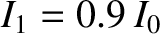takes the form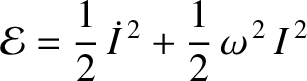(62)

where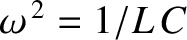is the mass,the spring force constant, anda constant (with the dimensions of angular frequency) that parameterizes the strength of the damping. The time evolution equation of the system thus becomes [cf., Equation (2)]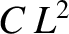(63)

where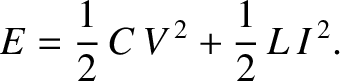is the undamped oscillation frequency [cf., Equation (6)]. We shall refer to the preceding equation as the damped harmonic oscillator equation.

Let us search for a solution to Equation (63) of the form(64)

where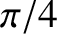,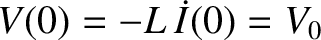,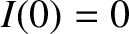, and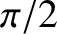are all constants. By analogy with the discussion in Section 2.1, we can interpret the preceding solution as a periodic oscillation, of fixed angular frequency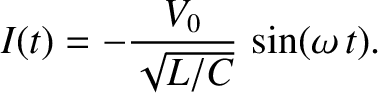, and phase angle, whose amplitude decays exponentially in time as. It can be demonstrated that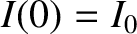(65)(66)

Hence, Equation (63) becomes

 0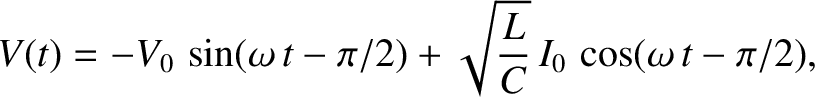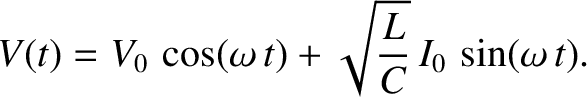(67)

The only way that the preceding equation can be satisfied at all times is if the (constant) coefficients of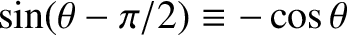and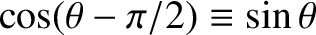separately equate to zero, so that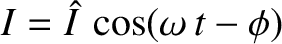(68)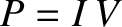(69)

These equations can be solved to give(70)

and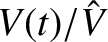(71)

Thus, the solution to the damped harmonic oscillator equation is written(72)

assuming that(because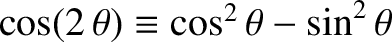cannot be negative). We conclude that the effect of a relatively small amount of damping, parameterized by the damping constant, on a system that exhibits simple harmonic oscillation about a stable equilibrium state is to reduce the angular frequency of the oscillation from its undamped value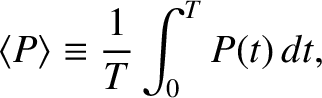to, and to cause the amplitude of the oscillation to decay exponentially in time at the rate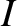. This modified type of oscillation, which we shall refer to as damped harmonic oscillation, is illustrated in Figure 7. [Here,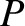,, and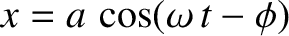. The solid line shows, whereas the dashed lines show.] Incidentally, if the damping is sufficiently large that(which we shall assume not to be the case) then the system does not oscillate at all, and any motion simply decays away exponentially in time. (See Exercise 7.)Although the angular frequency,, and decay rate,, of the damped harmonic oscillation specified in Equation (72) are determined by the constants appearing in the damped harmonic oscillator equation, (63), the initial amplitude,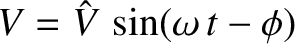, and the phase angle,, of the oscillation are determined by the initial conditions. In fact, if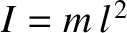and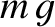then it follows from Equation (72) that(73)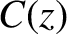(74)

giving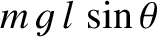(75)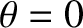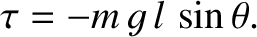(76)

The damped harmonic oscillator equation is a linear differential equation. In other words, ifis a solution then so is, whereis an arbitrary constant. It follows that the solutions of this equation are superposable, so that ifandare two solutions corresponding to different initial conditions thenis a third solution, whereand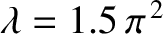are arbitrary constants.

Multiplying the damped harmonic oscillator equation, (63), by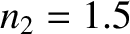, we obtain(77)

which can be rearranged to give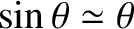(78)

where(79)

is the total energy of the system: that is, the sum of the kinetic and potential energies. Because the right-hand side of (78) cannot be positive, and is only zero when the system is stationary, the total energy is not a conserved quantity, but instead decays monotonically in time due to the action of the damping. The net rate at which the force (62) does work on the mass is(80)

The spring force (i.e., the first term on the right-hand side) does negative work on the mass (i.e., it reduces the system kinetic energy) whenandare of the same sign, and does positive work when they are of the opposite sign. It can be demonstrated that, on average, the spring force does no net work on the mass during an oscillation cycle. The damping force, on the other hand, (i.e., the second term on the right-hand side) always does negative work on the mass, and, therefore, always acts to reduce the system kinetic energy.Next: Quality Factor Up: Damped and Driven Harmonic Previous: Damped and Driven Harmonic
Richard Fitzpatrick 2013-04-08